Question

1. Evaluate the limit. (Use symbolic notation and fractions where needed. Enter "DNE" if limit does...

1. Evaluate the limit.

(Use symbolic notation and fractions where needed. Enter "DNE" if limit does not exist.)
lim : x→10

(x−10)/(x2−100)=

2. Evaluate the limit.

(Use symbolic notation and fractions where needed.)
lim : x→−6

(x^2+13x+42)/(x+6)=

3. Evaluate the limit:
lim : x→0

(cot7x)/(csc7x)=

4.Evaluate the limit.

(Use symbolic notation and fractions where needed. Enter "DNE" in answer field if limit does not exist.)

lim : x→1

[(7/(1−x)) −(14/(1−x^2))]=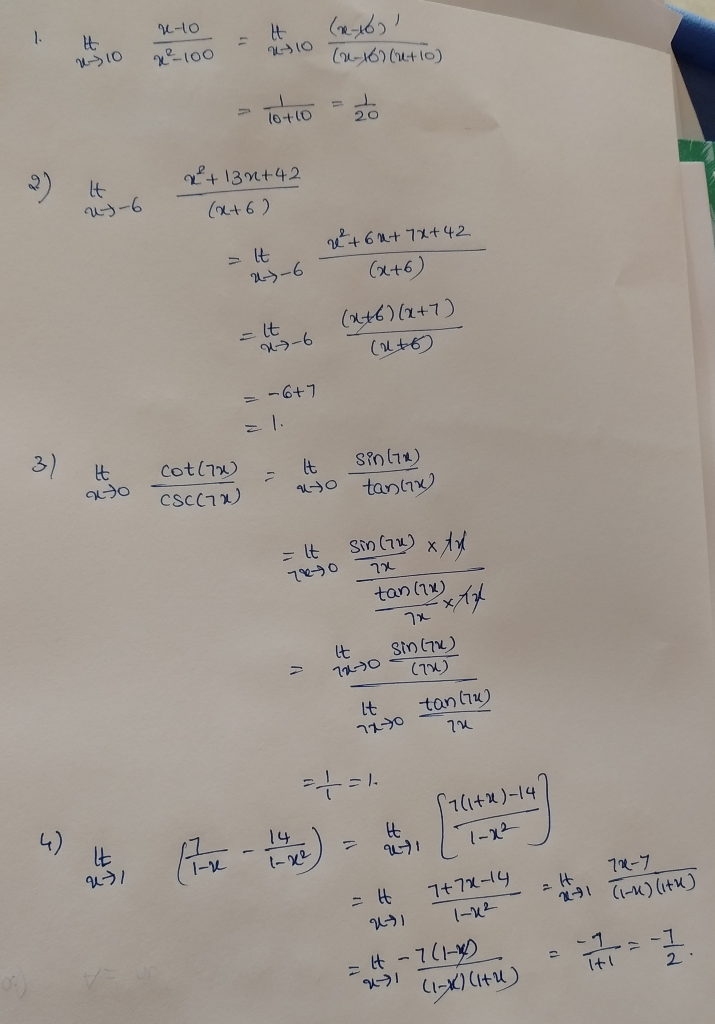Earn Coins

Coins can be redeemed for fabulous gifts.

Similar Homework Help Questions
• Find the Maclaurin series for f(x) = cos (x*). (Use symbolic notation and fractions where needed.)...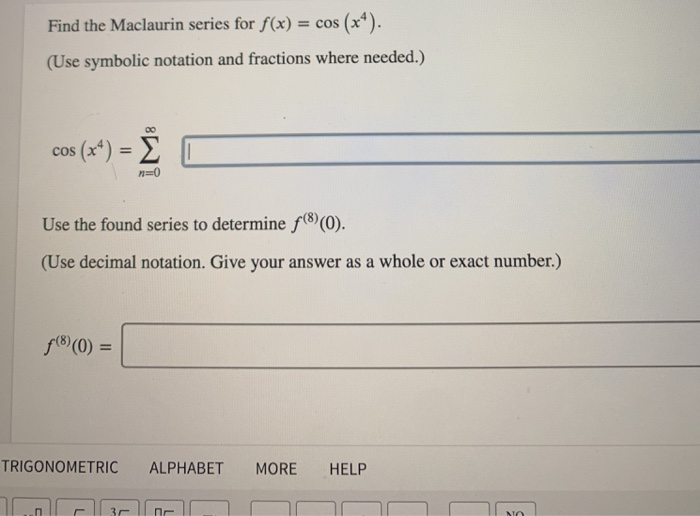Find the Maclaurin series for f(x) = cos (x*). (Use symbolic notation and fractions where needed.) cos (x4) = E O Use the found series to determine f(8)(0). (Use decimal notation. Give your answer as a whole or exact number.) f(8)(0) = TRIGONOMETRIC ALPHABET MORE HELP mn 4 of 6 > Compute the limit by substituting the Maclaurin series for the trig function. (Use symbolic notation and fractions where needed.). sin (9x) – 9x + 2 lim X-0

• Evaluate the limit. (If an answer does not exist, enter DNE.) lim 7 + et X-01-er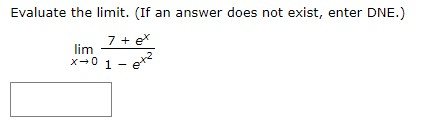Evaluate the limit. (If an answer does not exist, enter DNE.) lim 7 + et X-01-er

• Evaluate the limit, if it exists. (If an answer does not exist, enter DNE.) lim V...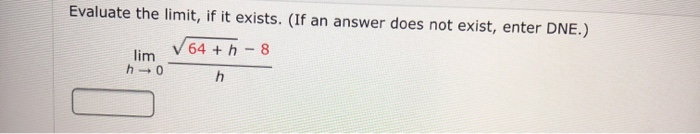Evaluate the limit, if it exists. (If an answer does not exist, enter DNE.) lim V 64 + - 8 h0

• Use properties of limits and algebraic methods to find the limit, if it exists. (If the limit is infinite, enter '∞' or '-∞', as appropriate. If the limit does not otherwise exist, enter DNE.)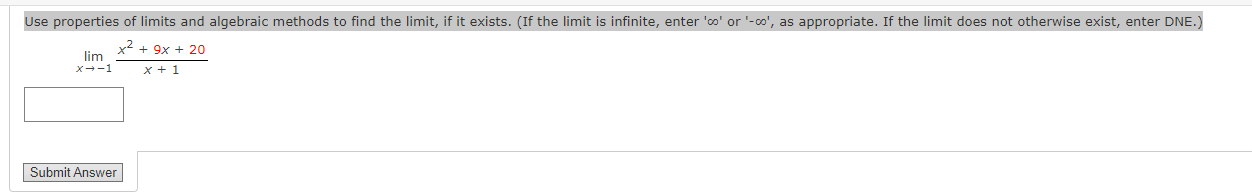Use properties of limits and algebraic methods to find the limit, if it exists. (If the limit is infinite, enter '∞' or '-∞', as appropriate. If the limit does not otherwise exist, enter DNE.)$$\lim _{x \rightarrow-1} \frac{x^{2}+9 x+20}{x+1}$$

• Solve the indefinite integral. Tv VX + 49 dx Use symbolic notation and fractions where needed....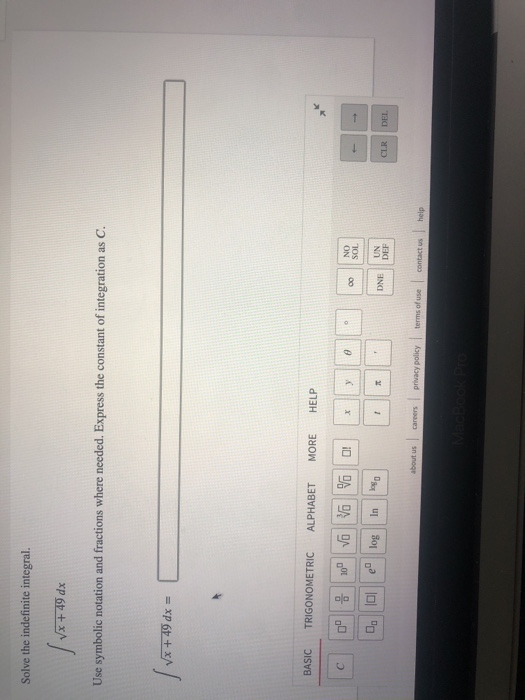Solve the indefinite integral. Tv VX + 49 dx Use symbolic notation and fractions where needed. Express the constant of integration as C. | vx +49dx = BASIC TRIGONOMETRIC ALPHABET MORE HELP с 10 vo vo v O! y o 00 NO SOL ! 0. 10 e log In 1 DNE UN DEF CLR DEL about us Careers privacy policy terms of use contact us help

• (1 point) Evaluate lim *+5 (x – 5)3 Enter I for 0,-1 for -00, and DNE...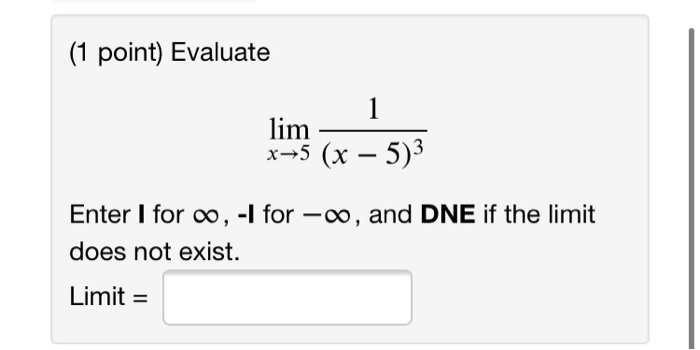(1 point) Evaluate lim *+5 (x – 5)3 Enter I for 0,-1 for -00, and DNE if the limit does not exist. Limit =

• Determine the limit of the sequence or state that the sequence diverges. an = 3 3...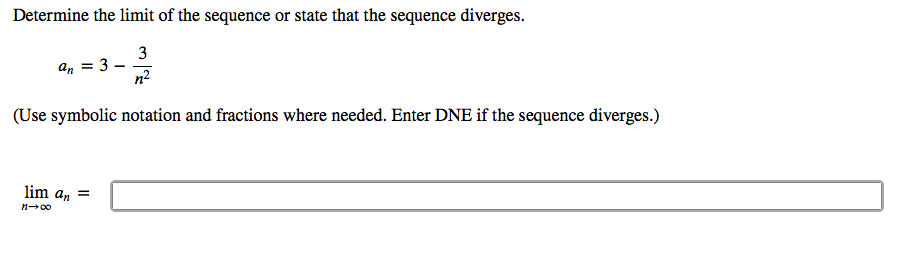Determine the limit of the sequence or state that the sequence diverges. an = 3 3 n2 (Use symbolic notation and fractions where needed. Enter DNE if the sequence diverges.) lim an = n-00

• evaluate without using a calculator. sin^-1(sin(-17pi/6)) (1 point) Evaluate without using a calculator. (Use symbolic notation...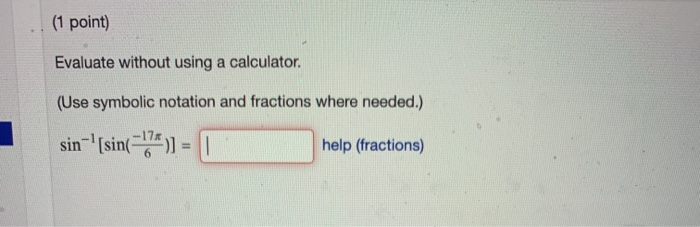evaluate without using a calculator. sin^-1(sin(-17pi/6)) (1 point) Evaluate without using a calculator. (Use symbolic notation and fractions where needed.) sin-' [sin(174) ) = 0 help (fractions)

• The graphs of f and g are given. Use them to evaluate each limit, if it exists. (If an answer does not exist, enter...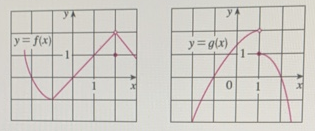The graphs of f and g are given. Use them to evaluate each limit, if it exists. (If an answer does not exist, enter DNE.) (a) $$\lim _{x \rightarrow 2}[f(x)+g(x)]$$(b) $$\lim _{x \rightarrow 1}[f(x)+g(x)]$$(c) $$\lim _{x \rightarrow 0}[f(x) g(x)]$$(d) $$\lim _{x \rightarrow-1} \frac{f(x)}{g(x)}$$(e) $$\lim _{x \rightarrow 2}\left[x^{3} f(x)\right]$$(f) $$\lim _{x \rightarrow 1} \sqrt{3+f(x)}$$

• Use the graph to determine the limit. (If an answer does not exist, enter DNE.)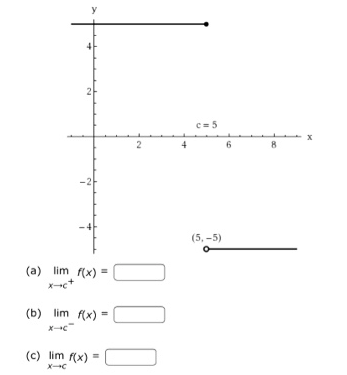Use the graph to determine the limit. (If an answer does not exist, enter DNE.) Is the function continuous at x=5 ?YesNo